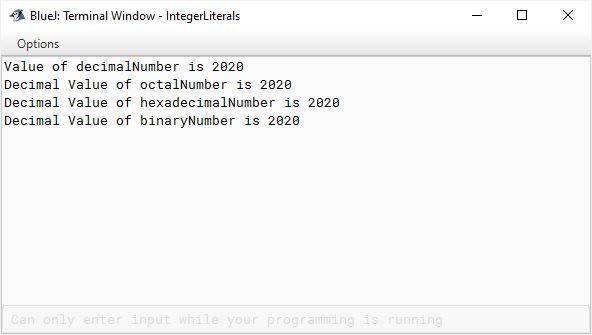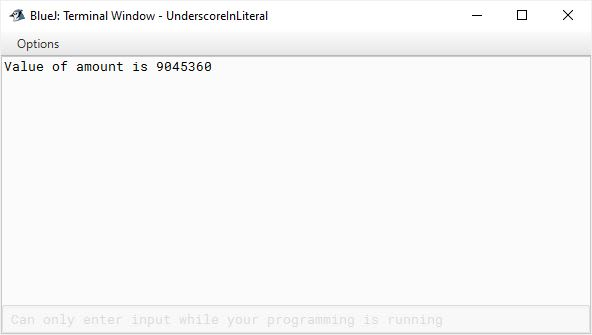# Integer Literals

## ICSE Computer Applications

I briefly touched upon Literals in the Code Blocks and Tokens lecture. Now is the time to dive deep into it. To quickly recap, any constant value which can be assigned to the variable is called a literal. Let's start by looking at integer literals.

Any whole number value is an integer literal. Integer literals are most commonly used literals in a typical program. `2`, `16`, `18426`, `24041973` are some examples of integer literals.

In our programs almost all of the times we use decimal numbers meaning base 10 numbers. In addition to decimal numbers, Java also supports Octal, Hexadecimal and Binary numbers.

### Octal (base 8)

To write a number as an Octal number prefix it with a `0`. Java considers numbers with leading zeros as Octal numbers. So, don’t prefix your number with a `0` if you don’t want it to be interpreted as an Octal number. Octal number system uses the digits 0 to 7. So, if you write `08` or `09` in your program, it will lead to an error as 8 and 9 are invalid Octal numbers.

To write a number as a Hexadecimal number, prefix it with a `0x`. The case of `x` doesn’t matter. You can use either `X` or `x`. Hexadecimal number system uses digits 0 to 9 to represent values from 0 to 9 and letters A to F to represents values from 10 to 15. Again, the case of letters A to F doesn’t matter you can use either lowercase or uppercase.

### Binary (base 2)

To write a number as a Binary number, prefix it with a `0b`. Here again, `b` can be in any case.

Let’s look at a program to understand all this with examples.

```public class NumberSystemDemo
{
public void demoNumberSystems() {

int decimalNumber = 2020;
System.out.println("Value of decimalNumber is "
+ decimalNumber);

//Octal representation of 2020
int octalNumber = 03744;
System.out.println("Decimal Value of octalNumber is " + octalNumber);

//Binary representation of 2020
int binaryNumber = 0b011111100100;
System.out.println("Decimal Value of binaryNumber is " + binaryNumber);
}
}```First, we declare an integer variable `decimalNumber` and assign it the value `2020` in base 10 and then print it. Nothing new here, we have been doing this since the beginning of this course.

Next, we declare an int variable `octalNumber`. Octal equivalent of decimal number `2020` is `3744`. We assign this octal value to the variable `octalNumber`. Notice, the leading `0` before `3744`. This tells Java that this integer literal is in base 8 or Octal form. We print the value of `octalNumber` variable in decimal form which comes out to `2020`.

After that, we declare an int variable `hexadecimalNumber`. Hexadecimal equivalent of decimal number `2020` is `7e4`. We assign this hexadecimal value to the variable `hexadecimalNumber`. Notice, the leading `0x` before `7e4`. This tells Java that this integer literal is in base 16 or hexadecimal form. The `println` statement prints `hexadecimalNumber` variable in decimal form which comes out to `2020`.

Next, we declare an int variable `binaryNumber` and to it we assign the binary equivalent of decimal number `2020`. We prefix the integer literal with `0b` which tells Java to treat it as a binary number not a decimal number. We then print the value of variable `binaryNumber` in decimal form which comes out to `2020`.

This should give you a good idea of how to use different number systems in Java.

### Underscores in Integer Literal

One interesting thing about integer literals is that you can embed one or more underscores in an integer literal. It will still be a valid literal, the underscores will be discarded at compile time. Underscores can come only in between the digits of the literal, they cannot come at the start or end of the literal. This is useful to improve the readability of the literal.

When we are writing currency amount, we use commas to separate the hundreds place, thousands place, etc. If you have to write an amount of ninety lakh forty-five thousand three hundred and sixty you will write it like this — 90,45,360. In Java, you cannot use commas in your integer literal. Instead you can use underscores and write it like this — 90_45_360. It will be a valid integer literal.

Let’s look at a BlueJ program to see this in action:

```public class UnderscoreDemo
{
public void demoUnderscore() {

int amount = 90_____45_____360;
System.out.println("Value of amount is " + amount);
}
}```You can add any number of underscores between the digits of an integer literal. All of them will be discarded and the integer literal will be printed without the underscores. So this is how you can use underscores to improve readability of your integer literals.